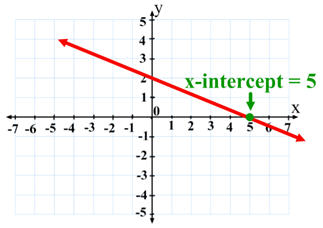# X-intercept & Y- intercept

The x-intercept is a point on the graph but on the other hand the y- intercept is a zero. the y- intercept is a point on the graph where the x becomes a zero. to calculate the x intercept you have to be using the formula (y-b), m=x, where the m equals the slope, and the y equals zero and the b equals the y-intercept. the y- intercept is the point which a linear equation crosses the y- axis on the x=0 plot point, the equation to determine both the slope and the y-intercept of a line is y=mx+b.